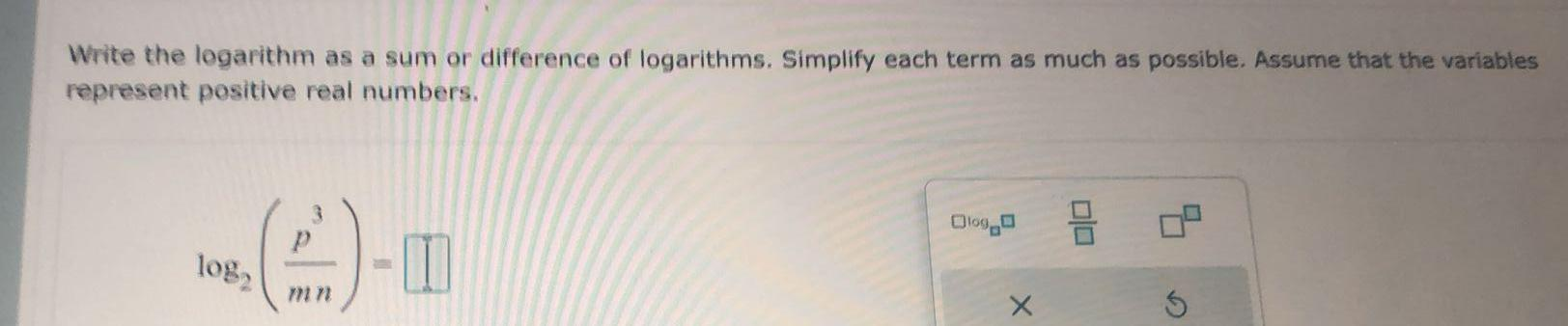Question:

# Write the logarithm as a sum or difference of logarithms.

Last updated: 9/1/2022Write the logarithm as a sum or difference of logarithms. Simplify each term as much as possible. Assume that the variables represent positive real numbers. log2(p3/mn)=